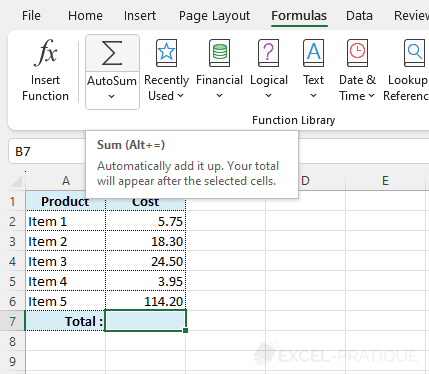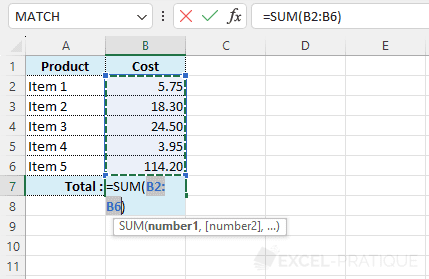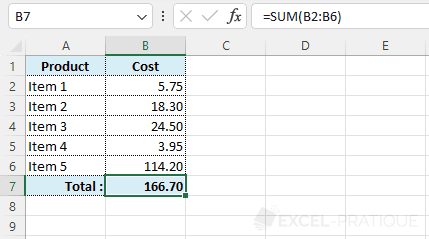# Excel Function: SUM

The Excel function SUM calculates the sum of a series of values.

Usage:

`=SUM(values)`

## Usage Example

The SUM function has its own shortcut, you don't need to search for SUM in the list of functions.

Click on the cell that will contain the sum and then on the "AutoSum" symbol ():A list of values is automatically preselected.

If it is correct, press Enter, if not, make another selection and then press Enter.The sum is calculated: